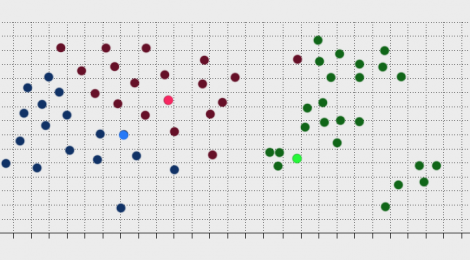## K-means clustering in simple words

Hello all.

Today I’ll attempt to explain in the most simple terms possible, what the k-means clustering algorithm does and how it can be used in your projects.

First I will attempt to give a simple intuition of the k-means process, then I will show you how to apply this knowledge to implement your own algorithm without looking at any code.

Let’s begin!

## What is a clustering algorithm?

I always think the best way to get an intuition of a hard concept is to start with an example and apply this knowledge to the theory.

Example time!

Immagine you have this set of points that need to divided into a 3 groups.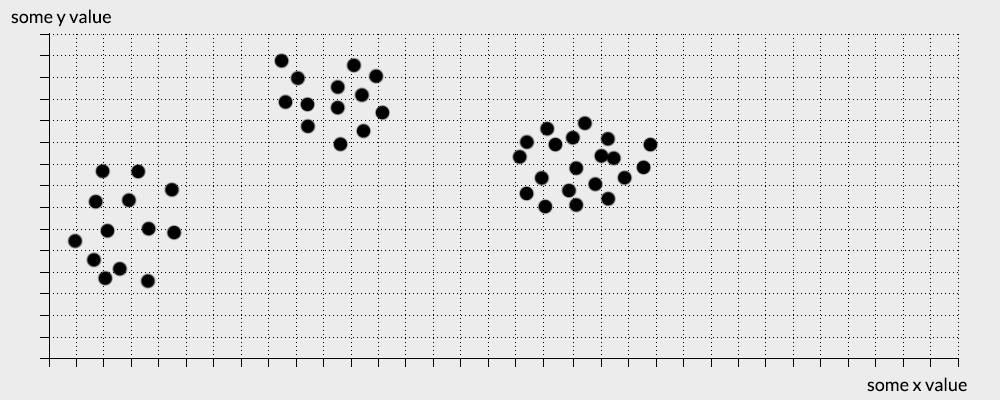As you can see for yourself, dividing this set is quite trivial and requires minimal mental effort. This is because the points in the data have either very similar values to other points or completely different ones. this forms natural clusters in the data.

Therefore you might divide the data set in this manner: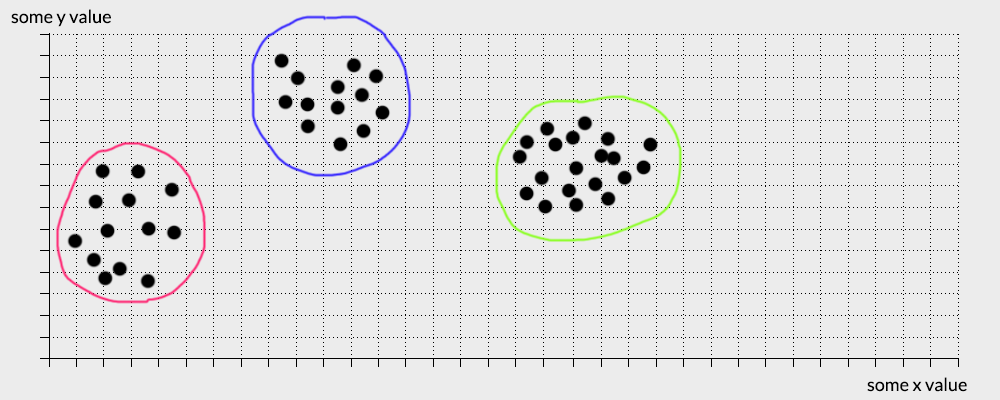If you divided the balls in this way, congratulations you just implemented the k-clustering algorithm… in your head (well kinda). Now you might be asking yourself, what is the point of this clustering algorithm. I just did this in my head, I don’t need an algorithm to do this.

Computer scientists have always created algorithms to make a once hard-to-solve task, simple and accessible to everyone, and so does the k-means clustering algorithm.

So when do we need to use this algorithm?

Now that you are feeling cocky, let’s see if you find this new challenge a little trickier (you will)

Try to divide this dataset into 5 groups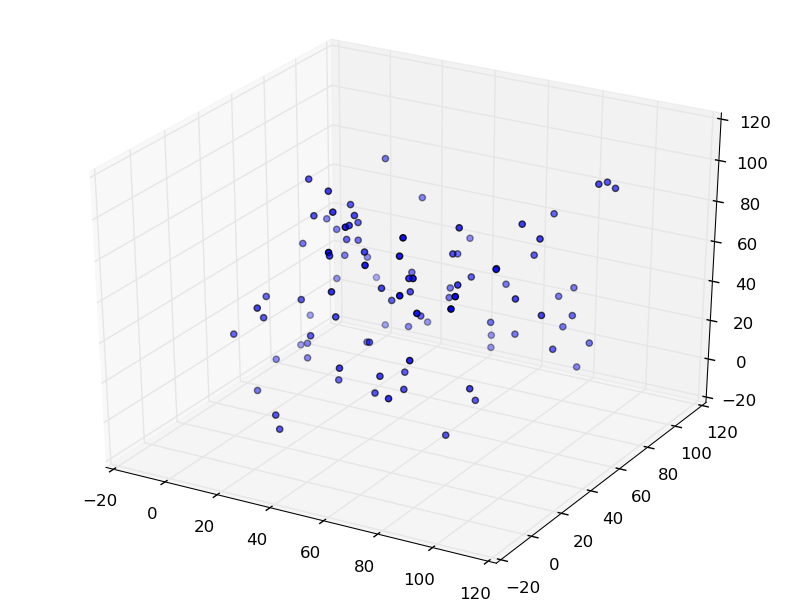Not so easy huh?

Given the x,y,z coordinates of these points, you might attempt to cluster these groups manually, but you will soon find that there seem to be infinite ways of clustering this dataset. Have you given up yet?

This is where clustering algorithms come into play. Clustering algorithms are extremely useful when we are trying to cluster complicated and high dimensional data. Where a human would fail spectacularly, clustering algorithms can provide us with extremely precise results. One very popular way to divide a dataset into clusters is by using the k-means clusters. Why is it called K means? Well because it uses K means 🙂

### How K-means clustering works

before we talk about the algorithm and code, let’s talk about how the general idea works with another example:

Lets consider this dataset. (lets keep it 2D for our brain’s health)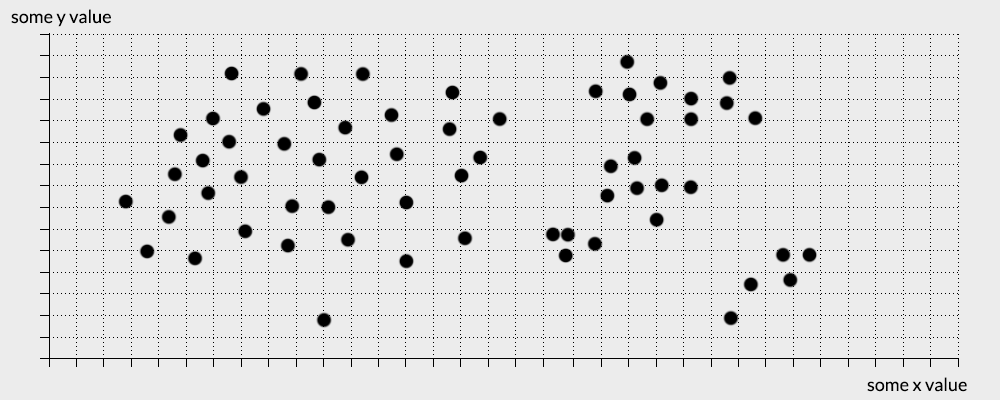1. The k-means algorithm forms empty clusters around randomly selected centres called centroids, in this example we set K=3.2. It then assigns each points to its nearest centroid based on their Euclidean distance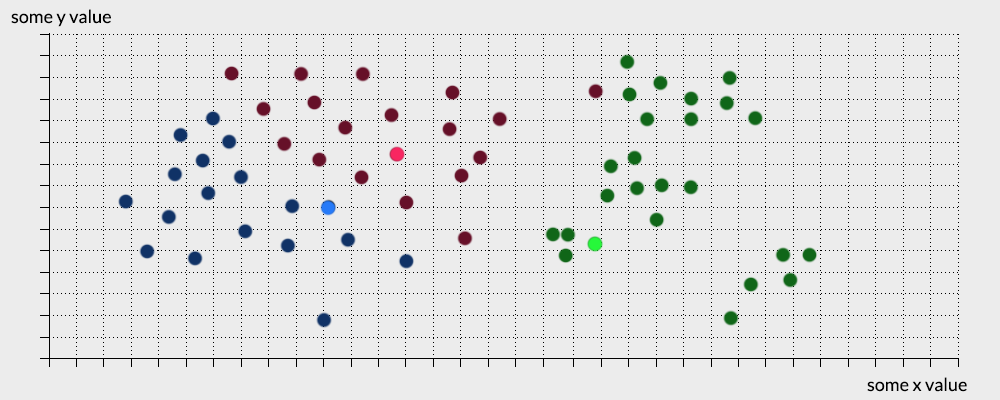3. It calculates the mean value of each cluster and updates the centroids based on this value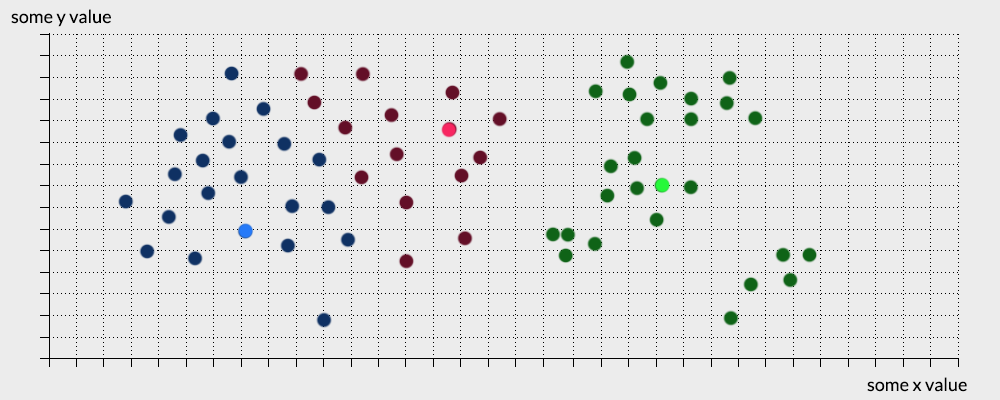4. Steps 2 and 3 are repeated until no changes are made to the centroids assignments

If you are still having difficulties in understanding this process, have a look at this fantastic visualisation by Naftali Harris

This algorithm is knows as a non deterministic algorithm, which means that the results will not always be the same for the same input data. In fact, depending on the random initialisation of the centroids, the clusters can change. As a rule of thumb, we can observe the clustered data, and if we notice that the results change dramatically with each run, this could be a suggestion that the data is not well fitted for clustering.

I hope this small post has given you some better intuition for the algorithm and how it works. Pleas have a go at implementing k-means on your own, i’m sure you won’t find it hard and the results are very satisfying.

If however, you do get stuck you can find many implementations of the code with a quick Google search 🙂